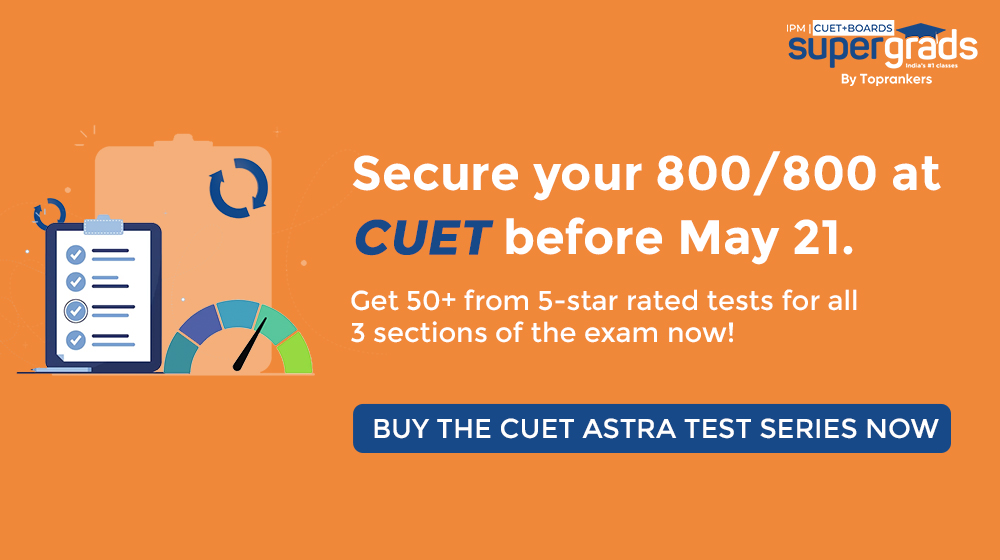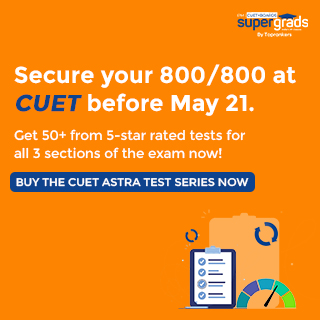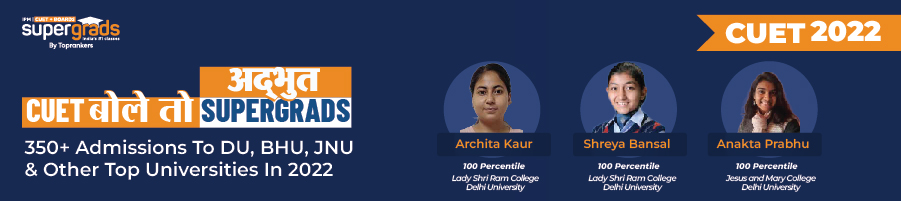• Home
• CUET Maths Syllabus ...

Author : Aparna

Updated On : September 17, 2023

SHARE

Summary: Several UG courses like B.Sc.(Hons.) Instrumentation, B.Tech, B.Com, etc., include maths in their exam pattern, which makes Mathematics preparations unavoidable for CUET admission. Since CUET was recently introduced, students might face issues with understanding or finding the right syllabus. Here's help!

Understanding the syllabus for any subject is an integral part of the preparation process to achieve excellent scores.

NTA has released the syllabus for Maths on the official website for the next academic year.

The syllabus is quite similar to that of last year. There are several books for Mathematics preparations; however, getting your basics right from NCERT textbooks will suffice for good preparations.

This article helps you with the CUET Mathematics Exam Syllabus 2024 as the NTA prescribes.

Section II has domain subjects, including Mathematics, and its syllabus is divided into 2 sections:

• Section- A
• Section B (Section B1 and Section- B2)

## CUET Maths Syllabus 2024 Highlights

The Central University Entrance Test (CUET 2024) will be administered to establish a level playing field for all applicants, irrespective of how well they performed in the class 12 board test.

The mathematics curriculum for CUET is quite comprehensive and is broken down into smaller sections for you.

The specific question format for each unit is broken down into the following categories:

• There will be one Question Paper which will contain Two Sections i.e. Section A and Section B [B1 and B2].
• Section A will have 15 questions covering both i.e. Mathematics/Applied Mathematics which will be compulsory for all candidates
• Section B1 will have 35 questions from Mathematics out of which 25 questions need to be attempted.
• Section B2 will have 35 questions purely from Applied Mathematics out of which 25 question will be attempted.## CUET Maths Syllabus 2024

The sections and topics under Mathematics CUET Exam Syllabus 2024 are elaborated as follows:

### Mathematics/ Applied Mathematics (319) Syllabus for class 12

Applied Mathematics topics to be prepared are as follows:

### Section B1: Mathematics Course

Unit I: Relations and Functions

1. Relationships and Roles

Topics: Forms of relationships: Reflexive, symmetric, transitive, and equivalence relations. One-to-one and onto functions, composite functions, and the function's inverse. The use of binary operations.

2. Trigonometric Inverse Functions

Topics: Definition, range, domain, major value branches. Inverse trigonometric function graphs. The fundamental characteristics of inverse trigonometric functions.

Unit II: Algebra

1. Matrices

Topics: Concept, notation, order, equality, matrices types, zero matrices, matrix transpose, symmetric matrices. Matrix addition, multiplication, and scalar multiplication; addition, multiplication, and scalar multiplication as basic characteristics. Noncommutativity of matrix multiplication and the presence of nonzero matrices whose product is zero matrices (restricted to square matrices of order 2).

Topics: Concept about elementary row and column operations Invertible matrices and, if possible, confirmation of the uniqueness of the inverse; (Here, all matrices will have real entries).

2. Determinants

Topics: Determinants of a square matrix (up to 3 3 matrices), their characteristics, minors, and applications in calculating the area of a triangle. Inverse and adjoint of a square matrix. Example of consistency, inconsistency, and several solutions of a system of linear equations; solution of a system of linear equations in two or three variables (with a unique solution) using the inverse of a matrix.

Unit III: Calibration

1. Continuity and Distinctiveness

Topics: Continuity and differentiability, the derivative of composite functions, the chain rule, the derivatives of inverse trigonometric functions, and the derivative of an implicit function. Exponential and logarithmic function concepts. Derivativesoflog x index. Differentiation is based on logarithmic differentiation. Derivative of parametrically expressed functions. Second-order differentiation. Rolle's and Lagrange's Mean Value Theorems and their geometric implications (without proof).

2. Derivative Applications

Topics: Rate of change, increasing/decreasing functions, tangents and normals, approximation, maxima, and minima are applications of derivatives (the first derivative test is motivated geometrically, and the second derivative test is given as a provable tool). Simple problems (that illustrate basic principles and understanding of the subject and real-life situations). Standard and Tangent

3. Three Applications of Integrals

Topics: Applications for determining the area under simple curves, including lines, arcs of circles/parabolas/ellipses (in standard form only), and the area between the two aforementioned curves (the region should be identifiable).

Check: CUET 2024 Mock Tests

Unit IV: Vectors and three-dimensional Geometry

1. Vectors

Topics: Vectors and scalars, vector magnitude and direction, Vector direction cosines and ratios. Vector types (equal, unit, zero, parallel, and collinear vectors), the position vector of a point, the negative of a vector, the components of a vector, the addition of vectors, the multiplication of a vector by a scalar, and the position vector of a point splitting a line segment in a certain ratio. Scalar product of vectors, vector projection onto a line. Vector (cross) product and vector triple product.

2. The study of three-dimensional geometry

Topics: Cosines and ratios of the direction of a line joining two locations. Cartesian and vector line equations, coplanar and skew lines, and the shortest distance between two lines. A plane's Cartesian and vector equations. The angle between two lines, planes, or a line and a plane. The distance between a point and a plane.

UNIT V: Linear Programming

Topics: Introduction, terminology such as constraints, objective function, and optimization, various types of linear programming (L.P.) problems, mathematical formulation of L.P. problems, graphical method of solution for two-variable problems, feasible and infeasible regions, feasible and infeasible solutions, optimal feasible solutions (up to three non-trivial constraints).

Check: CUET Coaching 2024

Unit VI: Probability

Topics: Multiplications in theorem regarding probability. Baye's theorem, conditional probability, independent events, and total probability. Random variable, its probability distribution, and its mean and standard deviation. Repeated independent trials (Bernoulli) and the Binomial distribution.### Section B2: Applied Mathematics

These are the units available for applied mathematics:

Unit I: Numbers, Quantity, and Numerical Applications

1. Modulo Arithmetic

Topics:

• Define the modulus of an integer
• Use modular arithmetic rules to perform arithmetic operations

2. Congruence Modulo

Topics:

• Define congruence modulo
• Apply the definition to several problems

3. Allegation and Combination

Topics:

• Determine the mean price of a mixture
• Comprehend the rule of allegation for producing a mixture at a particular price
• Apply the rule stated in the accusation

4. Numerical Problems

Topics:

• Solve mathematically real-world problems

5. Boats and Streams

Topics:

• Differentiate between upstream and downstream
• Write the problem as an equation

6. Pipes and cisterns

Topics: Calculate the time required for two or more pipes to fill or drain.

7. Contests and sports

Topics: Compare the performance of two players in terms of time, distance travelled, and work accomplished using the provided data.

8. Partnership

Topics:

• Distinguish between an active partner and a sleeping partner
• Calculate the gain or loss to be distributed among the partners based on the proportion of each partner's investment to the total investment
• evaluation of time/volume/surface area for solids created by combining two or more shapes

9. Numerical Inequalities

Topics:

• Describe the fundamental ideas of numerical inequalities
• Understand numerical inequalities and write them

Unit II: Algebra

These are the topics under Unit II:

1. Types of matrices and matrices

Topics:

• Define matrix
• Recognize many types of matrices

2. Equivalence of matrices Matrix transpose, symmetric and skew-symmetric matrix

Topics:

• Determine the equivalence of two matrices
• Write transpose of a given matrix
• Define symmetric and skewsymmetric matrix

Unit III: Calibration

These are the topics under Unit II:

1. Higher Order Derivatives

Topics:

• Calculate second and higher-order derivatives
• Understand the differentiation of parametric functions and implicit functions Identify dependent and independent variables

2. Marginal Revenue and Marginal Cost Using Derivatives

Topics:

• Determine marginal cost and revenue
• Find marginal cost and marginal revenue

3. Maxima and minima

Topics:

• Determine critical points of the function
• Find the point(s) of local maxima and local minima and the accompanying local maximum and local minimum values
• Determine the absolute maximum and absolute minimum value of a function

Check: Domain Subjects in CUET

Unit IV: Probability Distributions

1. Probability Distribution

Topics:

• Understand the idea of Random Variables and their Probability Distributions
• Find the probability distribution of the discrete random variable

2. Mathematical Expectation

Topics:

Use the arithmetic mean of the frequency distribution to determine the anticipated value of a random variable

3. Variance

Topics:

Calculate the Variance and Standard Deviation of a random variableUNIT V: Index Numbers and Time-Based Data

1. Index Numbers

Topics:  Define Index numbers as a special type of average

2. Development of index numbers

Topics: Create various types of index numbers

3. Test of Index Numbers' Adequacy

Topics: Apply the time reversal test

UNIT VI: Population and Statistics

1. Population and Sample

Topics:

• Define Population and Sample
• Differentiate between population and sample
• Define a representative sample from a population

2. Parameters and statistics, as well as Statistical Interferences

Topics:

• Define the Parameter of the Population
• Define Statistics about the Sample
• Explain the relationship between Parameters and Statistics
• Explain the limitation of Statistics to generalize the estimation of the Population
• Interpret the concept of Statistical Significance and Statistical Inferences
• State the Central Limit Theorem
• Explain the relationship between Population, Sampling Distribution, and Sample.

UNIT VII: Index Numbers and Time-Based Data

1. A.Time Series

Topics: Determine that time series are chronological data

2. Components of Time Series

Topics: Differentiate between distinct time series components

3. Time Series analysis for univariate data

Topics: Solve practical problems based on statistical data and Interpret

Check: CUET Sociology Syllabus

UNIT VIII: Financial Mathematics

1. Endowment and Sinking Funds

Topics:

• Define perpetuity and sinking fund
• Calculate perpetuity
• Distinguish between sinking fund and savings account.

2. Bond Valuation

Topics:

• Define the idea of bond valuation and related concepts
• Determine the bond's value using the present value method

3. EMI Calculation

Topics:

• Describe the notion of electromagnetic interference (EMI)
• Calculate EMI using various ways

4. Linear Depreciation Method

Topics:

• Define the idea of linear depreciation
• Interpret the cost, residual value, and usable life of an item based on the facts provided
• Calculate depreciation

UNIT IX: Linear Programming

1. Introduction and pertinent terms

Topics: Acquaint oneself with terms associated with Linear Programming Problem

2. B.Mathematicalformulation of Linear Programming Problem

Topics: Formulate Linear Programming Problem

3. Different Linear Programming Problem Types

Topics: Identify and develop various LPP kinds

4. Graphical Solution Method for Two-Variable Problems

Topics: Draw the graph for a system of linear inequalities involving two variables and graphically determine its solution

Check: CUET Economics Syllabus

5. Feasible and Infeasible Regions

Topics: Identify feasible, infeasible, and bounded regions

6. Feasible and impractical solutions, optimum feasible solution

Topics:

• Recognize feasible and impractical options
• Identify the optimal feasible solution

Note: You do not have to focus on Mathematics if the UG program you applied to has no Maths syllabus.

### Conclusion

Due to the extensive amount of practice required, mathematics is widely regarded as the most challenging topic among all the subjects. You cannot become an expert in the subject unless you frequently practice problems from the syllabus.

For this, it is necessary to have complete knowledge of the subjects to be covered in the curriculum. The preceding part of this article offers a detailed curriculum summary.

Practice several mocks and solve previous year's papers to study at top universities nationwide.

Get CUET Study Materials 2024 [Free]

What are some of the options available to me if I opt for Mathematics?How many universities provide mathematics programs that students may take that will count toward their CUET exam in 2024?I need to enhance my accuracy when responding to the questions for the CUET Exam 2024. What can I do?How exactly can I get started with my preparation for the CUET Exam 2024?What should I practise under Probability?